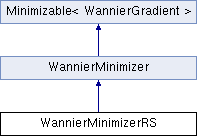JDFTx  1.7.0WannierMinimizerRS Class Reference

Wannier minimization with real-space localization measure. More...

#include <WannierMinimizerRS.h>

Inheritance diagram for WannierMinimizerRS:## Public Member Functions

WannierMinimizerRS (const Everything &e, const Wannier &wannier)

void initialize (int iSpin)
Prepare for minimization of spin channel iSpin.

Like getOmega, but for the subspace-invariant OmegaI instead.Public Member Functions inherited from WannierMinimizer
WannierMinimizer (const Everything &e, const Wannier &wannier, bool needSuperOverride=false)

void initTransformDependent ()
second half of common initialization that must happen after sub-class is fully initialized

void saveMLWF ()
save wannier functions for all spins

void saveMLWF (int iSpin)
save for specified spin

Move the state in parameter space along direction dir with scale alpha.

identity preconditioner, but impose hermiticity constraint

enforce hermiticity

bool report (int iter)

double sync (double x) const
All processes minimize together; make sure scalars are in sync to round-off error.

void bcastU ()Public Member Functions inherited from Minimizable< WannierGradient >
virtual double safeStepSize (const WannierGradient &dir) const
Override to return maximum safe step size along a given direction. Steps can be arbitrarily large by default.

double minimize (const MinimizeParams &params)
Minimize this objective function with algorithm controlled by params and return the minimized value.

void fdTest (const MinimizeParams &params)Protected Member Functions inherited from WannierMinimizer
bool isMine (size_t ik) const

int whose (size_t ik) const

bool isMine_q (int ik, int iSpin) const

int whose_q (int ik, int iSpin) const

ColumnBundle getWfns (const Kpoint &kpoint, int iSpin, std::vector< matrix > *VdagResultPtr=0) const
Get the wavefunctions for a particular k-point in the common basis (and optionally retrieve psp projections)

void axpyWfns (double alpha, const matrix &A, const Kpoint &kpoint, int iSpin, ColumnBundle &result, std::vector< matrix > *VdagResultPtr=0) const
Like getWfns, but accumulate instead of setting, and with optional transformation matrix: result += alpha * wfns * A.

void axpyWfns_grad (double alpha, matrix &Omega_A, const Kpoint &kpoint, int iSpin, const ColumnBundle &Omega_result) const
Gradient propagation corresponding to axpyWfns: from dOmega/d(result) to dOmega/dA.

matrix overlap (const ColumnBundle &C1, const ColumnBundle &C2, const std::vector< matrix > *VdagC1ptr=0, const std::vector< matrix > *VdagC2ptr=0) const

Preconditioner for Wannier optimization: identity by default, override in derived class to change.Static Protected Member Functions inherited from WannierMinimizer
static matrix fixUnitary (const matrix &U, bool *isSingular=0)Protected Attributes inherited from WannierMinimizer
const Everythinge

const Wannierwannier

const std::vector< SpaceGroupOp > & sym

int nCenters

int nFrozen

int nBands
number of Wannier centers (total and frozen) and source bands

int nSpins

int qCount
number of spins, and number of states per spin

int nSpinor
number of spinor components

std::vector< double > rSqExpect
Expectation values for r^2 per center in current group.

std::vector< vector3<> > rExpect
Expectation values for r per center in current group.

std::vector< bool > pinned
Whether centers are pinned or free.

std::vector< vector3<> > rPinned
Where centers are pinned to (if they are)

bool needSuper
whether supercell is necessary

GridInfo gInfoSuper
supercell grid

Basis basisSuper
supercell wavefcuntion basis

QuantumNumber qnumSuper
supercell k-point

int nPhononModes
number of phonon modes

diagMatrix invsqrtM
1/sqrt(M) per nuclear displacement mode

std::vector< KmeshEntrykMesh
k-point mesh with FD formula

std::set< Kpointkpoints
list of all k-points that will be in use (including those in FD formulae)

std::shared_ptr< ColumnBundleTransform::BasisWrapperbasisWrapper

std::shared_ptr< ColumnBundleTransform::BasisWrapperbasisSuperWrapper
look-up tables for initializing transforms

std::map< Kpoint, std::shared_ptr< ColumnBundleTransform > > transformMap

std::map< Kpoint, std::shared_ptr< ColumnBundleTransform > > transformMapSuper
wave-function transforms for each k-point to the common bases

Basis basis
common basis (with indexing into full G-space)

size_t ikStart

size_t ikStop

std::vector< ColumnBundleCother
wavefunctions from another process

std::vector< std::vector< matrix > > VdagCother
psp projections of wavefunctions from another process

## Detailed Description

Wannier minimization with real-space localization measure.

## ◆ getOmega()

 double WannierMinimizerRS::getOmega ( bool grad )
virtual

Return Omega and set rExpect and rSqExpect. If grad=true, set Omega_U in the KMeshEntry array. Note base class handles computing U and propagating gradients. At input, U will be available on all processes and Omega_U will be zero. Base class will accumulate Omega_U across processes on return.

Implements WannierMinimizer.

The documentation for this class was generated from the following file:
• wannier/WannierMinimizerRS.h